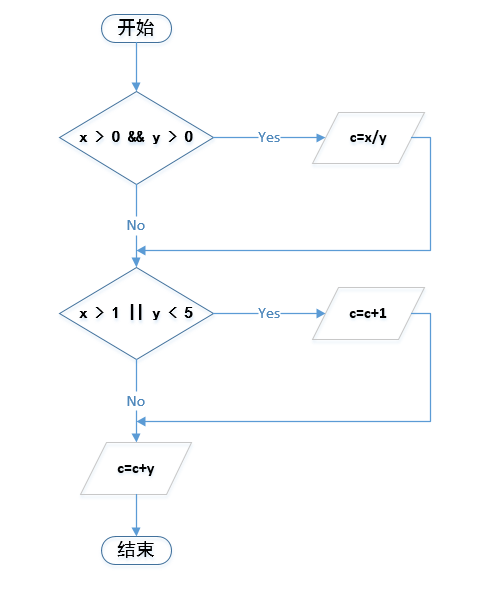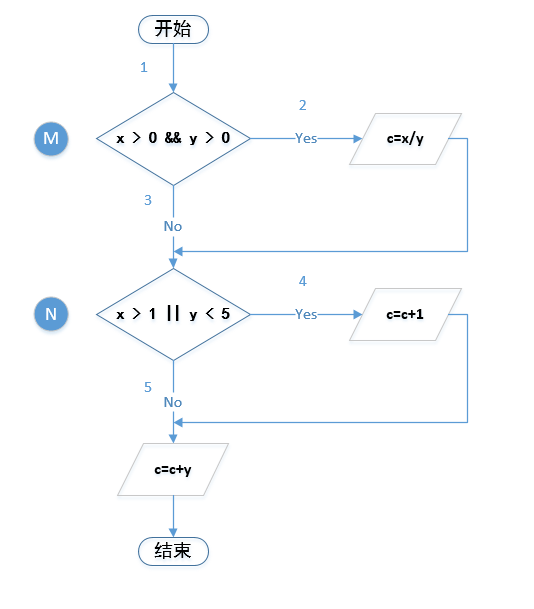# 设计方法

## 语句覆盖

• 如果是顺序结构，就让程序从头执行到尾
• 如果有分支，条件和循环，利用如下方法，执行足够的测试覆盖全部语句

### 设计用例`设计一条用例即可达到语句覆盖`

x = 2, y = 3

## 判定覆盖

### 设计用例x=2,y=4覆盖M-yes，N-yes
x=0,y=7覆盖M-no，N-no

## 条件覆盖### 设计用例

 判定条件 条件 取值 简称 M x>0 TRUE T1 FALSE F1 y>0 TRUE T2 FALSE F2 N x>1 TRUE T3 FALSE F3 y< 5 TRUE T4 FALSE F4

x=2,y=-2x>0,y<=0,x>1,y<5T1,F2,T3,T4
x=-1,y=6x<=0,y>0,x<=1,y>=5F1,T2,F3,F4

## 判定-条件覆盖

### 设计用例• 覆盖T1-T4 ，F1-F4
• 覆盖M为真/假,N为真/假

x=1,y=5x>0,y>0,x<=1,y>=5T1,T2,F3,F4M=T,N=F1-2-5
x=0,y=0x<=0,y<=0,x<=1,y<5F1,F2,F3,T4M=F,N=T1-3-4
x=2,y=-2x>0,y<=0,x>1,y<5T1,F2,T3,T4M=F,N=T1-3-4

## 条件组合覆盖

### 设计用例

1T1,T2M=Tx>0,y>0,M为真
2T1,F2M=Fx>0,y<=0.M为假
3F1,T2M=Fx<=0,y>0.M为假
4F1,F2M=Fx<=0,y<=0.M为假
5T3,T4N=Tx>1,y<5. N为真
6T3,F4N=Tx>1,y>=5,N为真
7F3,T4N=Tx<=1,y<5, N为真
8F3,F4N=Fx<=1,y>=5, N为假

x=2,y=4T1,T2,T3,T4M=T，N=T1，51-2-4
x=1,y=0T1,F2,F3,T4M=F,N=T2，71-3-4
x=0,y=6F1,T2,F3,F4M=F,N=F3，81-3-5
x=-1,y=-1F1,F2,F3,T4M=F,N=T4，71-3-4
x=4,y=5T1,T2,T3,F4M=F,N=T1，61-2-4

## 路径覆盖

### 设计用例

x=2,y=4T1,T2,T3,T4M=T，N=T1,51-2-4
x=1,y=0T1,F2,F3,T4M=F,N=T2,71-3-4
x=0,y=6F1,T2,F3,F4M=F,N=F3,81-3-5
x=-1,y=-1F1,F2,F3,T4M=F,N=T4,71-3-4
x=4,y=5T1,T2,T3,F4M=F,N=T1,61-2-4
x=0.5,y=3T1,T2,F3,F4M=T,N=F1,81-2-5